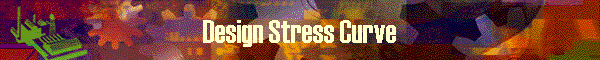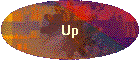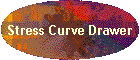This function allows users to design a new rod taper or modify the existing rod taper by drawing a Stress Curve.

It was difficult to specify a Stress Curve manually.  Especially it was the problem how to specify the small difference of Stress over the rod.

If it became true, we could establish a new method to define the target rod by drawing a Stress Curve.  DynaRod made it possible as its sub tool, "Stress Curve Drawer" which works on Excel workbook.  DynaRod and "Stress Curve Drawer" now co-works.  "Stress Curve Drawer" will define the target stress curve which is deflected.  DynaRod receives the stress distribution and calculate the dimension for the stress distribution.

DynaRod defines the word "Stress Curve" as follows;

In Garrison math, the stress curve is the plot of the number, the moment value divided by the section modulus, at each point over the rod.  This can also be said as "the plot of the stress values BEFORE the rod is deflected".

On the other hand, DynaRod's stress curve is the plot of the number, the moment value which is calculated reflecting the rod bend at the continuous point of time, divided by the section modulus, at each point over the rod.   This can also be said as "the plot of the stress values AFTER the rod is deflected".

User should be familiar with that the rod movement assumption, "Translated Rod", is used in the calculation of this function.

To design a completely new taper

As this program is so designed to start with a template rod in order to prepare the starting dimensions of a rod, user must select an existing rod as a template.

Then how to design a rod taper "from scratch"?

It is WHEN changing the deflection via changing the Stress graph on "Stress Curve Drawer" tool.  Just neglect the existing Stress Curve of template and newly define the Stress line on the tool.     [Design by Stress Curve] program in DynaRod receives the newly defined Stress data and calculate the newly designed dimensions as a completely new taper.

Data on screen

 Items Meaning Graph Name shows the name of displayed graph Tip Dimension at point 0 user can specify the point 0 dimension number to force the tip top dimension during the dimension calculation. To nullify the entered number, press [reset] button. The number can be switched between inch and mm by pressing [toggle unit] button.

Function Buttons

 Name Function Reset clears Tip Dimension at point 0  to null Target Stress Curve shows the stress curve of target rod specification Original Rod Stress Curve shows the comparison graph between target and original rod stress curves.  Red graph is original and Blue graph is the target rod. Design  Stress Curve invokes Stress Curve Drawer Tool to change or newly define the rod stress data. Prompt message is displayed as, "Starting up Stress Curve Drawer from now.  After completing your design, close Excel and save the file." then, press OK. screen is switched to Stress Curve Drawer Tool automatically. (if not, find Excel mark on task bar and click it) (work on Stress Curve Drawer) (Close Excel and save file) returns to this screen automatically. (if not, find the DynaRod on task bar and click it) prompt message is shown as; "We will import deflection data from Stress Curve Drawer workbook." then, press OK to continue. press [CALCULATE dimension] button CALCULATE dimension starts the calculation from deflection data to dimension. During the calculation prompt message as ; "No of Repeats:  x   Convergence Precision xxxxxxxx" press OK to continue. turns to [Comparison Graphs] for your review. Return to Archive returns to Archive screen